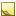﻿ IntegralImage Class# IntegralImage Class

Integral image.Inheritance Hierarchy
SystemObject
Accord.ImagingIntegralImage

Namespace:  Accord.Imaging
Assembly:  Accord.Imaging (in Accord.Imaging.dll) Version: 3.8.0Syntax
```[SerializableAttribute]
public class IntegralImage : ICloneable```

The IntegralImage type exposes the following members.Constructors
NameDescriptionIntegralImage
Initializes a new instance of the IntegralImage class.
TopProperties
NameDescriptionHeight
Height of the source image the integral image was constructed for.InternalData Obsolete.MatrixWidth
Width of the source image the integral image was constructed for.
TopMethods
NameDescriptionClone
Creates a new object that is a copy of the current instance.Equals
Determines whether the specified object is equal to the current object.
(Inherited from Object.)Finalize
Allows an object to try to free resources and perform other cleanup operations before it is reclaimed by garbage collection.
(Inherited from Object.)FromBitmap(Bitmap)
Construct integral image from source grayscale image.FromBitmap(BitmapData)
Construct integral image from source grayscale image.FromBitmap(UnmanagedImage)
Construct integral image from source grayscale image.GetHaarXWavelet
Calculate horizontal (X) haar wavelet at the specified point.GetHaarYWavelet
Calculate vertical (Y) haar wavelet at the specified point.GetHashCode
Serves as the default hash function.
(Inherited from Object.)GetRectangleMean(Int32, Int32, Int32)
Calculate mean value of pixels in the specified rectangle.GetRectangleMean(Int32, Int32, Int32, Int32)
Calculate mean value of pixels in the specified rectangle.GetRectangleMeanUnsafe(Int32, Int32, Int32)
Calculate mean value of pixels in the specified rectangle without checking it's coordinates.GetRectangleMeanUnsafe(Int32, Int32, Int32, Int32)
Calculate mean value of pixels in the specified rectangle without checking it's coordinates.GetRectangleSum(Int32, Int32, Int32)
Calculate sum of pixels in the specified rectangle.GetRectangleSum(Int32, Int32, Int32, Int32)
Calculate sum of pixels in the specified rectangle.GetRectangleSumUnsafe(Int32, Int32, Int32)
Calculate sum of pixels in the specified rectangle without checking it's coordinates.GetRectangleSumUnsafe(Int32, Int32, Int32, Int32)
Calculate sum of pixels in the specified rectangle without checking it's coordinates.GetType
Gets the Type of the current instance.
(Inherited from Object.)MemberwiseClone
Creates a shallow copy of the current Object.
(Inherited from Object.)ToString
Returns a string that represents the current object.
(Inherited from Object.)
TopFields
NameDescriptionintegralImage
Integral image's array.matrix
Integral image's array.
TopExtension Methods
NameDescriptionHasMethod
Checks whether an object implements a method with the given name.
(Defined by ExtensionMethods.)IsEqual
Compares two objects for equality, performing an elementwise comparison if the elements are vectors or matrices.
(Defined by Matrix.)To(Type)Overloaded.
Converts an object into another type, irrespective of whether the conversion can be done at compile time or not. This can be used to convert generic types to numeric types during runtime.
(Defined by ExtensionMethods.)ToTOverloaded.
Converts an object into another type, irrespective of whether the conversion can be done at compile time or not. This can be used to convert generic types to numeric types during runtime.
(Defined by ExtensionMethods.)
TopRemarks

This class implements integral image concept, which is described by Viola and Jones in: P. Viola and M. J. Jones, "Robust real-time face detection", Int. Journal of Computer Vision 57(2), pp. 137–154, 2004.

"An integral image I of an input image G is defined as the image in which the intensity at a pixel position is equal to the sum of the intensities of all the pixels above and to the left of that position in the original image."

The intensity at position (x, y) can be written as:

```          x    y
I(x,y) = SUM( SUM( G(i,j) ) )
i=0  j=0```Note
The class uses 32-bit integers to represent integral image.Note
The class processes only grayscale (8 bpp indexed) images.Note
This class contains two versions of each method: safe and unsafe. Safe methods do checks of provided coordinates and ensure that these coordinates belong to the image, what makes these methods slower. Unsafe methods do not do coordinates' checks and rely that these coordinates belong to the image, what makes these methods faster.

This class implements the simplest upright representation of an integral image. For an integral image that can represent squared integral images as well as tilted images at the same time, please refer to IntegralImage2.Examples

Sample usage:

```// create integral image
IntegralImage im = IntegralImage.FromBitmap(image);

// get pixels' mean value in the specified rectangle
float mean = im.GetRectangleMean(10, 10, 20, 30)```See Also